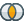You are viewing the RapidMiner Studio documentation for version 9.2 - Check here for latest version

#Intersect (RapidMiner Studio Core)

## Synopsis

This operator returns those examples of the first ExampleSet (given at the example set input port) whose IDs are contained within the other ExampleSet (given at the second port). It is necessary that both ExampleSets should have the ID attribute. The ID attribute of both ExampleSets should be of the same type.

## Description

This operator performs a set intersection on two ExampleSets on the basis of the ID attribute i.e. the resulting ExampleSet contains all the examples of the first ExampleSet (given at the example set input port) whose IDs appear in the second ExampleSet (given at the second port). It is important to note that the ExampleSets do not need to have the same number of columns or the same data types. The operation only depends on the ID attributes of the ExampleSets. It should be made sure that the ID attributes of both ExampleSets are of the same type i.e. either both are nominal or both are numerical.

## Differentiation

###Set Minus

The Set Minus and Intersect operators can be considered as opposite of each other. The Set Minus operator performs a set minus on two ExampleSets on the basis of the ID attribute i.e. the resulting ExampleSet contains all the examples of the first ExampleSet whose IDs do NOT appear in the second ExampleSet.

## Input

•example set input (IOObject)

This input port expects an ExampleSet. It is the output of the Generate ID operator in the attached Example Process because this operator only works if the ExampleSets have the ID attribute.

•second (IOObject)

This input port expects an ExampleSet. It is the output of the Generate ID operator in the attached Example Process because this operator only works if the ExampleSets have the ID attribute.

## Output

•example set output (IOObject)

The ExampleSet with remaining examples (i.e. examples remaining after the set intersection) of the first ExampleSet is output of this port.

•original (IOObject)

The ExampleSet that was given as input (at example set input port) is passed without changing to the output through this port. This is usually used to reuse the same ExampleSet in further operators or to view the ExampleSet in the Results Workspace.

## Tutorial Processes

### Intersection of two ExampleSets

The 'Golf' data set is loaded using the Retrieve operator. The Generate ID operator is applied on it with the offset parameter set to 0. Thus the ids of the 'Golf' data set are from 1 to 14. A breakpoint is inserted here so you can have a look at the 'Golf' data set. The 'Polynomial' data set is loaded using the Retrieve operator. The Generate ID operator is applied on it with the offset parameter set to 10. Thus the ids of the 'Polynomial' data set are from 11 to 210. A breakpoint is inserted here so you can have a look at the 'Polynomial' data set.

The Intersect operator is applied next. The 'Golf' data set is provided at the example set input port and the 'Polynomial' data set is provided at the second port. The order of ExampleSets is very important. The Intersect operator compares the ids of the 'Golf' data set with the ids of the 'Polynomial' data set and then returns only those examples of the 'Golf' data set whose id is present in the 'Polynomial' data set. The 'Golf' data set ids are from 1 to 14 and the 'Polynomial' data set ids are from 11 to 210. Thus 'Golf' data set examples with ids 11 to 14 are returned by the Intersect operator. It is important to note that the meta data of both ExampleSets is very different but it does not matter because the Intersect operator only depends on the ID attribute.

If the ExampleSets are switched at the input ports of the Intersect operator the results will be very different. In this case the Intersect operator returns only those examples of the 'Polynomial' data set whose id is present in the 'Golf' data set. The 'Golf' data set ids are from 1 to 14 and the 'Polynomial' data set ids are from 11 to 210. Thus the 'Polynomial' data set examples with ids 11 to 14 are returned by the Intersect operator.